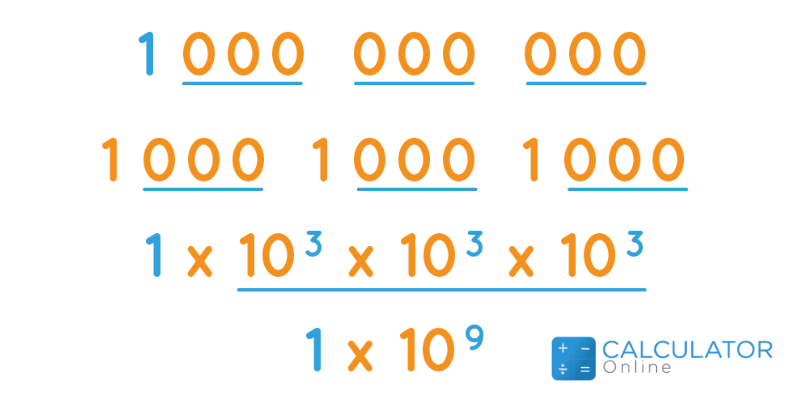Uh Oh! It seems you’re using an Ad blocker!

We always struggled to serve you with the best online calculations, thus, there's a humble request to either disable the AD blocker or go with premium plans to use the AD-Free version for calculators.

Or# How do You Write Billion in Numbers?

## How do You Write Billion in Numbers?

One billion equals 10,000 lakhs which is further equal to 1,000,000,000. Generally, 1 billion in numbers consists of a large number of significant digits in the place value. The value 999,999,999 comes before one billion in number and the 1,000,000,001 comes after that number. In mathematics, the concept of place value is used to indicate quantities. There are multiple ways to analyze the place values but the International System of Units (SI) is the best way to interpret the place value of the digits in a number. However, the place value technique is widely used to describe the number's positional values. Numbers can be represented in general form with the help of positional values.

### How to Write 1 Billion in Numbers?

Sometimes you need to write one billion in number for specific reasons. Regardless of the reasons, we explain a method of how to write billion in numbers. Let's find:
• To convert 1 billion in number form, first of all, multiply 1 by 10^9. Another way to write a billion in numbers is to move the decimal separator 9 places such as 1 × 10^9 = 1000000000
• Also, 1000000000 is separated by 1 = 10 = 100 = 1,000 = 10,000 = 100,000 = 1,000,000 = 10,000,000 = 100,000,000 = 1,000,000,000.
• 1 billion in digits is represented as 1000000000. And, 1000000000 can be written in figures with thousand separators like 1,000,000,000.

### How to Write a Billion in Numeric Form?

In this section, we evaluate one billion in number form.
As you know 1 billion in number is equal to 1,000,000,000. Furthermore, one billion means:
• 1 × 10^9 = 1000000000 as an integer.

### How do you Write 1 Billion in Numbers (cardinal or ordinal)?

In language, one billion in numbers can be written as either a cardinal numeral or an ordinal numeral.
The number 1000000000 indicates a cardinal number that is used to represent quantities.
On the other hand, the value of 1000000000th shows the ordinal number to express rank in sequential order or position.
Example 1:
I wish I had 1,000,000,000 dollars to spend on my marriage. This condition shows that it is a cardinal number.
Example 2:
The teacher tells the students that the 1000000000th part of 1B equals 1. It indicates that the given value expresses an ordinal number.

### Billion in Numbers Example:

The given example will assist you to clarify your concept about billions in numbers.

Example:

What is the value of 7 billion in numbers?

Solution:

As you know 1 billion in numbers is equal to 1,000,000,000
Therefore, the value of 7 billion in numbers can be determined as follows:

7 Billion = 7 x 1,000,000,000

7 Billion = 7,000,000,000 in number